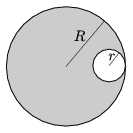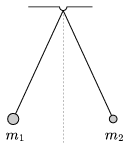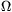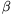Mathematical and Physical Journal
for High Schools
Issued by the MATFUND Foundation
 Already signed up? New to KöMaL?

# KöMaL Problems in Physics, October 2012

Show/hide problems of signs:## Problems with sign 'M'

Deadline expired on November 12, 2012.

M. 326. Hang a horse-shoe or a bar magnet above a compass, and rotate the magnet slowly by 360o, and observe the changes of the compass. Carry out the experiment at different distances between the compass and the magnet, and describe the behaviour of the compass.

(6 pont)

statistics## Problems with sign 'P'

Deadline expired on November 12, 2012.

P. 4465. Why can your breath be seen in cold weather?

(3 pont)

solution (in Hungarian), statistics

P. 4466. The trolley pole of electric train engines - big current flows through them - often sparks. The same current flows through the wheels into the rails as well. Why doesn't the wheels spark?

(3 pont)

solution (in Hungarian), statistics

P. 4467. A police car parking on the hard shoulder of a highway, is passed by a car at a speed of 108 km/h. (This is the greatest speed at which this car can travel.) After a short time it turns out in the police car that the passing car is a stolen one. The police car starts to chase the car ten seconds after the stolen car passed it. The maximum speed of the four-wheel drived police car is 162 km/h. The coefficient of static friction between the road and the wheels is 0.4. In order not to reach the traffic in the city it would be good to catch the escaping car in a distance of 1.5 km. Is it possible?

(4 pont)

solution (in Hungarian), statistics

P. 4468. A circular piece of radius r is cut out of a disc of radius R as shown in the figure. At what ratio of r/R will the centre of mass of the remaining piece be closer to the centre of the disc than R/100?(4 pont)

solution (in Hungarian), statistics

P. 4469. Two balls of masses m1 and m2 are hanged at the same point, with threads of equal lengths, and displaced towards the left and the right with the same angle measured from the vertical. They are both released at the same moment, and collide totally inelastically. The positions of the balls are described by their height above the horizontal plane which is through the lowest point of their paths. (Air resistance is negligible.) Give the ratio of the greatest height reached by the balls after the collision to the initial height.(4 pont)

solution (in Hungarian), statistics

P. 4470. The containers of A and B are connected by a short and thin tube. The volume of container A is three times as much as that of container B. Initially in both containers there is air at a temperature of 20 oC and at a pressure of 105 Pa. Then container A is heated to a temperature of 100 oC, and is kept at this temperature while the temperature of B is still 20 oC. By what percent does the mass of the gas in each container change, and what will the pressure be in them?

(4 pont)

solution (in Hungarian), statistics

P. 4471. Two batteries of voltage 3 V is connected in parallel. Their internal resistances are 3, and 2. Then an external resistor of resistance 10is connected to the batteries. What will the terminal voltage and the current through the batteries be?

(4 pont)

solution (in Hungarian), statistics

P. 4472. There are two coils wined around the same closed iron core. Their inductances on this iron core are measured to be 4 henry, and 9 henry. Then the two coils are connected in series. What will the equivalent inductance be?

(5 pont)

solution (in Hungarian), statistics

P. 4473. 0.06 mass percent of the human body is potassium, and 0.012% of this is the potassium isotope of atomic mass number 40. This isotope is radioactive, itdecays, and its half life is 1.3 miliard years. How manydecays occur in each second in the body of a 60-kg man due to the potassium in the human body?

(4 pont)

solution (in Hungarian), statistics

P. 4474. Estimate the size of that Aluminium ball which can stay at rest in the Solar system. Where can it stay at rest? (Neglect the effect of planets and moons and the particle currents in the Solar system.) The solar luminosity is 3.8.1026 W, its mass is 1.989.1030 kg, and the density of Aluminium is 2700 kg/m3.

(5 pont)

solution (in Hungarian), statistics